Trams

Two trams started at the same time from the same place. One tram journey takes 30 minutes and the second 45 minutes to its final stop. How long will trams meet again?

Result

t =  180 min

Solution:Leave us a comment of this math problem and its solution (i.e. if it is still somewhat unclear...):Be the first to comment!To solve this verbal math problem are needed these knowledge from mathematics:

Do you want to calculate least common multiple two or more numbers?

Next similar math problems:

1. Tram lines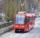Trams of five lines driven at intervals of 5,8,10,12 and 15 minutes. At 12 o'clock come out of the station at the same time. About how many hours again all meet? How many times have earch tram pass for this stop?
2. Runners circle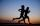Pepa circles the track in 36 seconds. Kamil in 42 seconds. They started together. How many seconds will meet again at the start?
3. Ships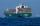Red ship begins its circuit every 30 minutes. Blue boat begins its circuit every 45 minutes. Both ships begin their sightseeing circuit in the same place at the same time always at 10:00 o'clock. a / What time does meet boat again? b / How many times a da
4. Gearing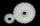Gearing consists of two wheels, one is 88 and second 56 teeth. How many times turns smaller wheel to fit the same teeth as in the beginning? How many times will turn biggest wheel?
5. Greg and BillGreg is 18 years old. He is 6 less than 4 times Bill's age. How old is Bill?
6. Masons1 mason casts 30.8 meters square in 8 hours. How long casts 4 masons 178 meters square?
7. Grandfather and grandmotherThe old mother is 5 years younger than the old father. Together they are 153 years old. How many years has each of them?
8. Class 9AOn the final certificate have one quarter of the class 9A mark "C" of mathematics, seventh mark "C" from the Czech language and two students failed in chemistry. How many students attend class 9A?
9. Raffle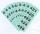In the pool is numbers from 1 to 115. What is the probability that a randomly selected number is not a prime number?
10. The percentages in practiceIf every tenth apple on the tree is rotten it can be expressed by percentages: 10% of the apples on the tree is rotten. Tell percent using the following information: a. in June rained 6 days b, increase worker pay 500 euros to 50 euros c, grabbed 21 fro
11. Plum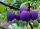On the platter are plum. How many were there if its have to be able to share equally among 8,10 and 12 children?
12. Lcm simpleFind least common multiple of this two numbers: 140 175.
13. Balls groups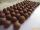Karel pulled the balls out of his pocket and divide it into the groups. He could divide them in four, six or seven, and no ball ever left. How little could be a ball?
14. MO 2016 Numerical axisCat's school use a special numerical axis. The distance between the numbers 1 and 2 is 1 cm, the distance between the numbers 2 and 3 is 3 cm, between the numbers 3 and 4 is 5 cm and so on, the distance between the next pair of natural numbers is always in
15. Conference148 is the total number of employees. The conference was attended by 22 employees. How much is it in percent?
16. Sales offGoods is worth € 70 and the price of goods fell two weeks in a row by 10%. How many % decreased overall?
17. Percents - easyHow many percent is 432 out of 434?